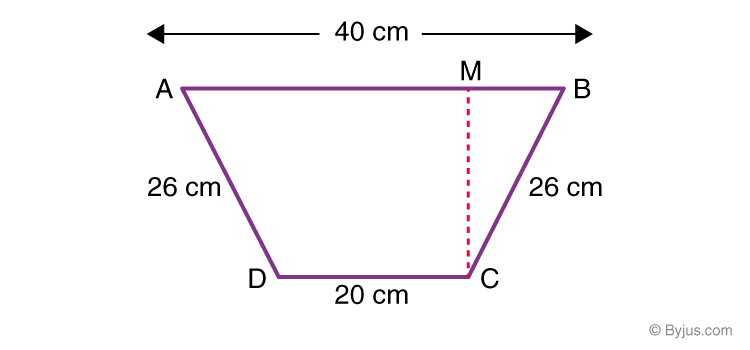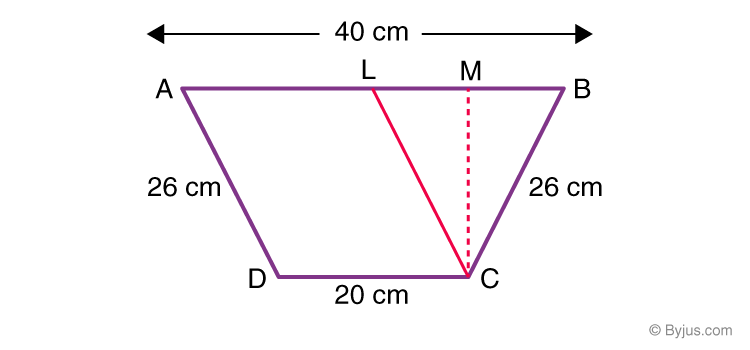# Important Questions Class 8 Maths Chapter 11 Mensuration

Important questions of Class 8 Maths Chapter 11 Mensuration are given here with answers for students. The questions are prepared as per the latest exam pattern and syllabus (2022-2023) by our subject experts. These Maths questions cover several short answer type questions, long answer type questions and HOTS (Higher Order Thinking Skills) questions that are crucial for CBSE exams. Here, some of the important mensuration questions from NCERT Class 8 are also included.

Also Check:

## Mensuration Important Questions For Class 8 (Chapter 11)

These Class 8 mensuration questions are categorized into short answer type questions and long answer type questions. These questions cover various concepts which will help students to develop problem-solving skills for the exam.

1. The parallel sides of a trapezium measure 12 cm and 20 cm. Calculate its area if the distance between the parallel lines is 15 cm.

Solution:

Area of trapezium = ½ × perpendicular distance between parallel sides × sum of parallel sides

= ½ × 15 × (12 + 20)

= 1/2 × 15 × 32

= 15 × 16

= 240  cm2

2. Calculate the height of a cuboid which has a base area of 180 cm2 and volume is 900 cm3.

Solution:

Volume of cuboid = base area × height

900 = 180 × height

So, height = 900/180 = 5 cm

3. A square and a rectangle have the same perimeter. Calculate the area of the rectangle if the side of the square is 60 cm and the length of the rectangle is 80 cm.

Solution:

Perimeter of square formula = 4 × side of the square

Hence, P (square) = 4 × 60 = 240 cm

Perimeter of rectangle formula = 2 × (Length + Breadth)

Hence, P (rectangle) = 2 (80 + Breadth)

= 160 + 2 × Breadth

According to the given question,
160 + 2 × Breadth = 240 cm
2 × Breadth = 240 – 160
The breadth of the rectangle = 40 cm

Now, the area of rectangle = Length × Breadth = 80 × 40 = 3200 cm2

4. A lawnmower takes 750 complete revolutions to cut grass on a field. Calculate the area of the field if the diameter of the lawnmower is 84 cm and the length is 1 m.

Solution:

Given, length of lawnmower = 1m = 100cm

Its circumference = π × D = 22/7 × 84 = 264 cm

Length of field will be = 264 × 750 = 198000 cm

Here, the width of field = length of the lawnmower i.e. 100 cm

So, area of field = 198000 × 100 = 19,800,000 cm²

Or, 1980 m²

5. The area of a rhombus is 16 cm2 and the length of one of its diagonal is 4 cm. Calculate the length of other diagonal.

Solution:

Area of rhombus = ½ × d1 × d2

⇒ 16 = ½ × 4 × d2

So, d2 = 32/4 = 8 cm

6. From a circular sheet of radius 4 cm, a circle of radius 3 cm is cut out. Calculate the area of the remaining sheet after the smaller circle is removed.

Solution:

The area of the remaining sheet after the smaller circle is removed will be = Area of the entire circle with radius 4 cm – Area of the circle with radius 3 cm

We know,

Area of circle = πr²

So,

Area of the entire circle = π(4)² = 16π cm2

And,

Area of the circle with radius 3 cm which is cut out = π(3)² = 9π cm2

Thus, the remaining area = 16π – 9π = 7π cm2

7. A cuboidal box of dimensions 1 m × 2 m × 1.5 m is to be painted except its bottom. Calculate how much area of the box has to be painted.

Solution:

Given,

Length of the box, l = 2 m,

Breadth of box, b = 1 m

Height of box, h = 1.5 m

We know that the surface area of a cuboid = 2(lb + lh + bh)

But here the bottom part is not to be painted.

So,

Surface area of box to be painted = lb + 2(bh + hl)

= 2 × 1 + 2 (1 × 1.5 + 1.5 × 2)

= 2 + 2 (1.5 + 3.0)

= 2 + 9.0

= 11

Hence, the required surface area of the cuboidal box = 11 m2

8. In a trapezium, the parallel sides measure 40 cm and 20 cm. Calculate the area of the trapezium if its non-parallel sides are equal having the lengths of 26 cm.

Solution:

From the question statement draw the diagram.

Consider a trapezium of ABCD. Let AB and DC be the parallel sides as shown in the figure.Now, CM will be the distance between the two parallel sides or the height of the trapezium.

We know,

Area of trapezium = ½ × sum of parallel sides × height.

So, height has to be found.

In the diagram, draw CL || ADNow, ALCD is a parallelogram ⇒ AL = CD = 20 cm and CL = AD = 26 cm

CL = CB ⇒ ΔCLB is an isosceles triangle with CB as its height.

Here, BL = AB – AL = (40 – 20) = 20 cm. So,

LM = MB = ½ BL = ½ × 20 = 10 cm

Now, in ΔCLM,

CL2 = CM2 + LM2 (Pythagoras Theorem)

262 = CM2 + 102

CM2 = 262 – 102

Using algebraic identities, we get; 262 – 10= (26 – 10) (26 + 10)

hence,

CM2 = (26 – 10) (26 + 10) = 16 × 36 = 576

CM = √576 = 24 cm

Now, the area of trapezium can be calculated.

Area of trapezium, ABCD = ½ × (AB + CD) × CM

= ½ × (20 + 40) × 24

Or, Area of trapezium ABCD = 720 cm2

## Class 8 Maths Chapter 11 Mensuration Extra Questions

1. A flooring tile is in the shape of a parallelogram with 24 cm base and the corresponding 10 cm height. Calculate the number of tiles required to cover a floor of area 1080 m(If required you can split the tiles in whatever way you want to fill up the corners).
2. Two cubes are joined end to end. Now, calculate the volume of the resulting cuboid, if each side of the cubes is 6 cm.
3. How many bricks each 25 cm by 15 cm by 8 cm, are required for a wall 32 m long, 3 m high and 40 cm thick?
4. Find the area of a rhombus whose one side measures 5 cm and one diagonal as 8 cm.

### More Topics Related to Class 8 Mensuration:

Visit BYJU’S to get more materials based on Class 8 and prepare for upcoming exams.

1. Thanks for sending these important questions. These will help me to attend this government exam. Thanks a lot. I already had joined in your app. Byjus app is more helpful for me. I am improving more. Thanks for this.

2. THANK YOU VERY MUCH FOR GIVING THE ABOVE PRACTICE PROBLEMS

3. THANKS FOR HELPING US.

4. So nice and helpful. Thanks a lot BYJU!

5. Thanks for these questions

6. thank u very much !!!!! 🙂

7. Thank u very much for these practice questions BYJUS! Thanks a lot ☺☺

8. Thank you for important questions that will help in my exam’s

9. Thank you so much for this important question it has helped me a lot